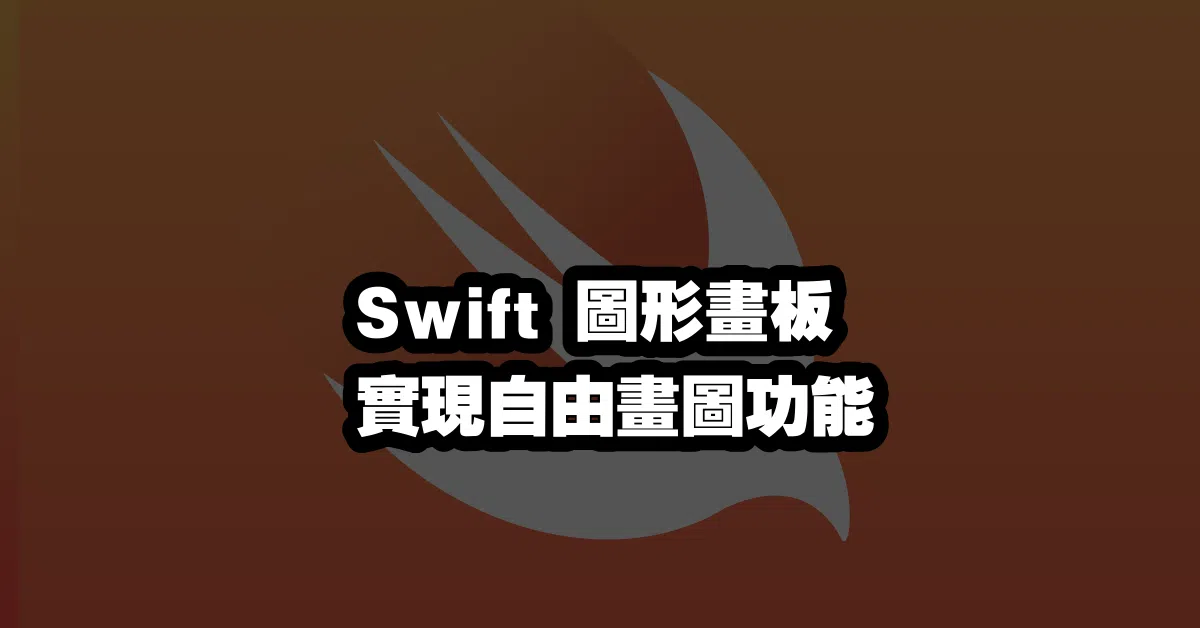# Swift 圖形畫板 🎨 實現自由畫圖功能

## Swift 圖形畫板 🎨 實現自由畫圖功能

``````let circle = UIBezierPath(ovalIn: CGRect(x: 0, y: 0, width: 100, height: 100))
let shapeLayer = CAShapeLayer()
shapeLayer.path = circle.cgPath
``````

``````let path = UIBezierPath()
path.move(to: CGPoint(x: 0, y: 0))
let shapeLayer = CAShapeLayer()
shapeLayer.path = path.cgPath